﻿ 基于不同核支持向量机变权综合的短期负荷预测 Short-Term Load Forecasting Based on Variable Weighted Synthesis of Different Kernel SVM

Statistics and Application
Vol. 09  No. 01 ( 2020 ), Article ID: 34113 , 8 pages
10.12677/SA.2020.91009

Short-Term Load Forecasting Based on Variable Weighted Synthesis of Different Kernel SVM

Dongfen Ma

School of Statistics and Data Science, Xinjiang University of Finance and Economics, Urumqi Xinjiang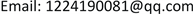Received: Jan. 17th, 2020; accepted: Jan. 31st, 2020; published: Feb. 6th, 2020ABSTRACT

To improve the accuracy and stability of short-term load forecasting, a method of short-term load forecasting based on different kernel support vector machine (SVM) variable weight synthesis is proposed. In this method, firstly, the load history data is expanded, the feature is selected by correlation analysis, and the historical data is mapped to the input-output relationship view to build the forecasting space. Then, support vector machines of Gaussian kernel, Laplace kernel and Polynomial kernel function are used to study in the forecasting space respectively, and the performance of the model is tested by the 10 fold cross validation. Finally, the variable weight is constructed by using the accuracy and standard deviation of performance test, and the power load forecasting is realized by variable weight synthesis of multiple model. Example analysis shows that compared with methods such as Gaussian kernel support vector machine, partial least squares, decision tree and Bagging, the new method improves accuracy by 0.382%, 3.079%, 3.188% and 2.6%, and stability by 0.383%, 2.452%, 1.781% and 1.43%, respectively.

Keywords:Short-Term Load Forecasting, SVM, Kernel Function, Variable Weight Synthesis of Multiple Models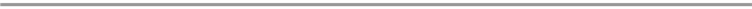1. 引言

2. 支持向量机算法原理

$\begin{array}{l}\mathrm{min}\frac{1}{2}{‖\omega ‖}^{2}+C\underset{i=1}{\overset{n}{\sum }}\left({\xi }_{i},{\xi }_{i}{}^{\ast }\right)\\ \left\{\begin{array}{l}{y}_{i}-〈\omega ,\phi \left({x}_{i}\right)〉-b\le \epsilon +{\xi }_{i},\text{}i=1,\cdots ,n;\\ 〈\omega ,\phi \left({x}_{i}\right)〉+b-{y}_{i}\le \epsilon +{\xi }_{i}{}^{\ast },\text{}i=1,\cdots ,n.\\ {\xi }_{i},{\xi }_{i}{}^{\ast }\ge 0\end{array}\end{array}$ (1)

$\begin{array}{l}L=\frac{1}{2}{‖\omega ‖}^{2}+C\underset{i=1}{\overset{n}{\sum }}\left({\xi }_{i},{\xi }_{i}{}^{\ast }\right)-\underset{i=1}{\overset{n}{\sum }}\left({\eta }_{i}{\xi }_{i},{\eta }_{i}{}^{\ast }{\xi }_{i}{}^{\ast }\right)\\ \text{}-\underset{i=1}{\overset{n}{\sum }}{\alpha }_{i}\left(\epsilon +{\xi }_{i}-{y}_{i}+〈\omega ,\phi \left({x}_{i}\right)〉+b\right)\text{}\\ \text{}-\underset{i=1}{\overset{n}{\sum }}{\alpha }_{i}^{\ast }\left(\epsilon +{\xi }_{i}{}^{\ast }-{y}_{i}+〈\omega ,\phi \left({x}_{i}\right)〉+b\right)\end{array}$ (2)

$f\left(x\right)=\underset{i=1}{\overset{n}{\sum }}\left({\stackrel{^}{\alpha }}_{i}-{\stackrel{^}{\alpha }}_{i}^{\ast }\right)k\left(x,{x}_{i}\right)+\stackrel{^}{b}$

3. 基于不同核支持向量机变权综合的短期负荷预测方法

3.1. 主要思路

3.2. 基于相关分析的负荷特征选择

${r}_{i}=r\left({f}_{0},{f}_{i}\right)=\frac{|\underset{k=1}{\overset{m}{\sum }}\left({s}_{t,k}-\frac{1}{m}\underset{k=1}{\overset{m}{\sum }}{s}_{t,k}\right)\left({s}_{ti,k}-\frac{1}{m}\underset{k=1}{\overset{m}{\sum }}{s}_{ti,k}\right)|}{\sqrt{\underset{k=1}{\overset{m}{\sum }}{\left({s}_{t,k}-\frac{1}{m}\underset{k=1}{\overset{m}{\sum }}{s}_{t,k}\right)}^{2}}\sqrt{\underset{k=1}{\overset{m}{\sum }}{\left({s}_{ti,k}-\frac{1}{m}\underset{k=1}{\overset{m}{\sum }}{s}_{ti,k}\right)}^{2}}}$ (3)

${r}_{i}$ 的取值为${r}_{i}$ 越接近于1， ${f}_{0}$${f}_{i}$ 之间的相关度越强。设定阈值 $\alpha$，将满足条件的特征所组成的特征集合表示为 ${F}_{t}^{\alpha }:\left\{{f}_{i}|{r}_{i}\ge \alpha \right\}$

3.3. 预测空间构造

${f}_{0}$ 为输出特征，以特征集 ${F}_{t}^{a}$ 内的特征为输入特征，建立预测空间 $\Omega =\underset{i=1}{\overset{n}{\prod }}X\left({f}_{i}\right)×X\left({f}_{0}\right)$

$D=\left\{\left({X}_{t},{s}_{t}\right),t=1,\cdots ,N\right\}$，其中 ${X}_{t}=\left({s}_{t-1},{s}_{t-2},\cdots ,{s}_{t-n}\right)$，N为关系化数据记录条数。

3.4. 核函数选择及模型建立

${k}_{G\text{-}K}\left(u,v\right)=\mathrm{exp}\left(-\gamma {‖u-{v}^{\text{T}}‖}^{2}\right)$ (4)

${k}_{P\text{-}K}\left(u,v\right)={\left(\gamma 〈u,v〉+c\right)}^{p}$ (5)

${k}_{La\text{-}K}\left(u,v\right)=\mathrm{exp}\left(-\gamma ‖u-{v}^{\text{T}}‖\right)$(6)

3.5. 模型性能测试及变权构造

${A}_{i}=1-{E}_{i}=1-\frac{1}{H}\underset{h=1}{\overset{H}{\sum }}{\epsilon }_{ih}=1-\frac{1}{H}\underset{h=1}{\overset{H}{\sum }}\left(\frac{|{y}_{ih}-{\stackrel{^}{y}}_{ih}|}{{y}_{ih}}\right)$ (7)

${\sigma }_{i}=\sqrt{\frac{1}{H}\underset{h=1}{\overset{H}{\sum }}{\left[\left(1-{\epsilon }_{hi}\right)-{A}_{i}\right]}^{2}}$ (8)

${w}_{k}=\frac{\underset{i=1}{\overset{P}{\sum }}{A}_{ki}\left(1-{\sigma }_{ki}\right)}{\underset{k=1}{\overset{3}{\sum }}\underset{i=1}{\overset{P}{\sum }}{A}_{ki}\left(1-{\sigma }_{ki}\right)}$ (9)

${\stackrel{^}{y}}^{*}=\underset{k=1}{\overset{3}{\sum }}{w}_{k}{\stackrel{^}{y}}_{k}$

3.6. 基于不同核函数SVM变权综合的负荷预测

Step 1：确定给定的历史数据集的特征展开参数m，将给定时刻t前m个负荷数据组成电力负荷特征向量 ${X}_{t}$$t=1,2,\cdots ,N-m$

Step 2：设待预测时刻 $t={t}_{z}$$z=1,\cdots ,Z$，确定负荷预测备选特征集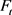，采用相关度分析进行特征选取，组建特征集 ${F}_{t}^{\alpha }$，进而对历史数据集进行关系化展开，构建待预测时刻的预测空间 $\Omega$

Step 3：选用高斯径向基核函数、拉普拉斯核函数及多项式核函数的支持向量机在预测空间上建立预测模型，借助P折交叉验证进行模型测试。

Step 4：依据性能测试结果的准确度 ${A}_{i}$ 及其标准差 ${\sigma }_{i}$ 构造变权，通过变权综合不同核函数的SVM实现 ${t}_{z}$ 的负荷预测。令 $z=z+1$，当 $z>Z$，则预测结束；否则，转Step 2。

4. 算例分析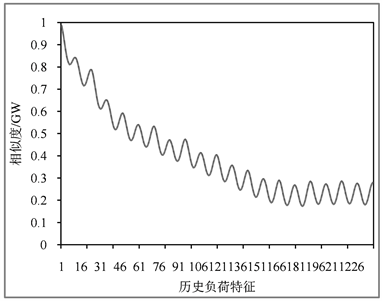Figure 1. Correlation between load feature to be forecast and historical load features

$MAPE=\frac{1}{n}\underset{i=1}{\overset{n}{\sum }}\frac{|{y}_{i}-{\stackrel{^}{y}}_{i}|}{{y}_{i}}×100%$ (10)

$SRE=\sqrt{\frac{1}{n}\underset{i=1}{\overset{n}{\sum }}{\left(\frac{|{y}_{i}-{\stackrel{^}{y}}_{i}|}{{y}_{i}}×100%-MAPE\right)}^{2}}$ (11)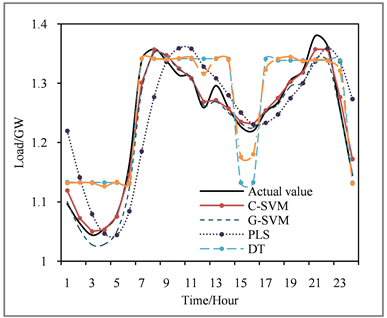Figure 2. Comparison of power load forecasting values

5. 总结

Short-Term Load Forecasting Based on Variable Weighted Synthesis of Different Kernel SVM[J]. 统计学与应用, 2020, 09(01): 73-80. https://doi.org/10.12677/SA.2020.91009

1. 1. 康重庆, 夏清, 刘梅. 电力系统负荷预测[M]. 北京: 中国电力出版社, 2017, 1-17.

2. 2. Khan, A.R., Mahmood, A., Safdar, A., et al. (2016) Load Forecasting, Dynamic Pricing and DSM in Smart Grid: A Review. Renewable & Sustainable Energy Reviews, 54, 1311-1322. https://doi.org/10.1016/j.rser.2015.10.117

3. 3. 顾熹, 廖志伟. 基于相空间重构和高斯过程回归的短期负荷预测[J]. 电力系统保护与控制, 2017, 45(5): 73-79.

4. 4. Chen, J.F., Wang, W.M. and Huang, C.M. (1995) Analysis of an Adaptive Time-Series Autoregressive Moving-Average (ARMA) Model for Short-Term Load Forecasting. Electric Power Systems Research, 34, 187-196. https://doi.org/10.1016/0378-7796(95)00977-1

5. 5. 张伏生, 汪鸿, 韩悌, 等. 基于偏最小二乘回归分析的短期负荷预测[J]. 电网技术, 2003, 27(3): 36-40.

6. 6. 万坤, 柳瑞禹. 区间时间序列向量自回归模型在短期电力负荷预测中的应用[J]. 电网技术, 2012, 36(11): 77-81.

7. 7. 魏立兵, 赵峰, 王思华. 基于人群搜索算法优化参数的支持向量机短期电力负荷预测[J]. 电测与仪表, 2015, 53(8): 45-49.

8. 8. Zhang, X.B., Wang, J.Z. and Zhang, K.Q. (2017) Short-Term Electric Load Forecasting Based on Singular Spectrum Analysis and Support Vector Machine Optimized by Cuckoo Search Algorithm. Electric Power Systems Research, 146, 270-285. https://doi.org/10.1016/j.epsr.2017.01.035

9. 9. 梁智, 孙国强, 等. 基于VMD与PSO优化深度信念网络的短期负荷预测[J]. 电网技术, 2018, 42(2): 598-606.

10. 10. 吴润泽, 包正睿, 宋雪莹, 等. 基于深度学习的电网短期负荷预测方法研究[J]. 现代电力, 2018, 35(2): 43-48.

11. 11. 杨芳君, 王耀力, 王力波, 等. 基于改进CS算法优化Elman-IOC神经网络的短期负荷预测[J]. 电测与仪表, 2019, 56(9): 39-44.

12. 12. Sudheer, G. and Suseelatha, A. (2015) Short Term Load Forecasting Using Wavelet Transform Combined with Holt-Winters and Weighted Nearest Neighbor Models. International Journal of Electrical Power & Energy Systems, 64, 340-346. https://doi.org/10.1016/j.ijepes.2014.07.043

13. 13. Li, S., Goel, L., and Wang, P. (2016) An Ensemble Approach for Short-Term Load Forecasting by Extreme Learning Machine. Applied Energy, 170, 22-29. https://doi.org/10.1016/j.apenergy.2016.02.114

14. 14. Wang, L., Lee, E.W.M. and Yuen, R.K.K. (2019) Novel Dynamic Forecasting Model for Building Cooling Loads Combining an Artificial Neural Network and an Ensemble Approach. Applied Energy, 228, 1740-1753. https://doi.org/10.1016/j.apenergy.2018.07.085

15. 15. 陆继翔, 张琪培, 等. 基于CNN-LSTM混合神经网络模型的短期负荷预测方法[J]. 电力系统自动化, 2019, 43(8): 191-197.